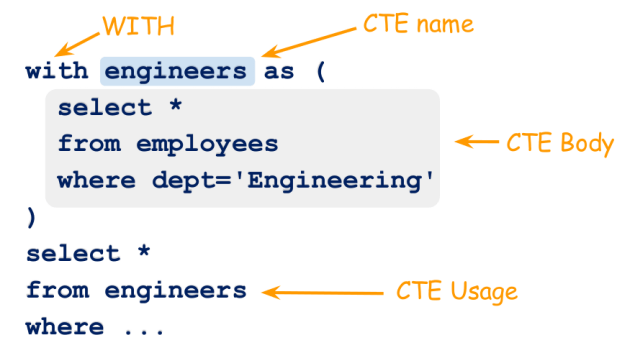# Solving the problem using Common Table ExpressionsHello devs 👋! This article is solely written for Django devs i.e, it is assumed you have an basic understanding on Django and used simple Django queries. But also other devs can also make use of the benefits of CTEs in a lot of scenearios.

## So what is CTE?

Few databases provides a way to store temporary results in a label(named dataset), that can be reused in the following queries. There are tons of articles written to explain CTE, but still here’s my part.

When I was explaining the same to my good old friend about Common Table Expression, she was like, “I’ll just use derived table for this. Why CTE?”. So, yes! There is a benefit of using CTE over derived table.

Let’s check the following derived table approach.

``````SELECT *
FROM (SELECT id, name FROM students where category == 'engineering')
as EngineeringStudents;``````

Now let us see how the sample above implemention can be approached using CTE.

``````WITH EngineeringStudents(id, name) AS (
SELECT *
FROM (SELECT id, name FROM students where category == 'engineering')
)

SELECT * FROM EngineeringStudents;``````

You might be asking, what is happening other than 2 extra lines in CTE? might also be with a rolling eyes.

The improvement over the first and latter is the readability and the reusability. Wondering how that is possible? Incase of multiple joins and some complex queries, you can easily do a magic like the one below.

``````WITH EngineeringStudents(id, name) AS (
SELECT *
FROM (SELECT id, name FROM students where category == 'engineering')
)
SELECT es1.id, es2.id FROM EngineeringStudents as es1
INNER JOIN EngineeringStudents as es1
ON es1.id = es2.parent_id
WHERE es1.parent_id = NULL;``````

If you really wanted me to explain the above SQL, you might need to brush up your SQL skills forehand.

So, yes! In few cases, CTE is really a friendly choice to take.

### Now taking it to our Django part

I was working on an application where we have Categories and these categories have subcategories and the depth goes on.

The python (fake) model looks like below.

``````class Category(models.Model):
name = models.CharField(max_length=150, blank=True)
parent = models.ForeignKey('Category', null=True, blank=True)``````

Now, given a category, I wanted to print all of it’s subcategories and their subcategories and so on. i.e, I wanted to print their descendants.

Don’t judge my code below. That was my naivest approach.

``````def get_descendants(root_category):
categories = []
for category in root_category.category_set.all():
categories.extend(get_descendants(category))
return categories``````

Do you know the number of DB calls this function was making? A lot. Too many. If the depth of categories are M and the width is N, our function was making M+N calls to DB. I was like 😭

### To the rescue

Then CTE came to the rescue. CTE has an awesome way to handle this problem using WITH RECURSIVE. You can read more on this here https://www.postgresql.org/docs/9.1/queries-with.html

I’ll share the code snippet with you, and you guys do homework on that. (Not explaining this. Because this is your homework. Still, you can DM me on this and I can explain you if you are really really interested.)

``````categories = Category.objects.raw('''
WITH RECURSIVE parent_category(id, parent_id) AS (
SELECT category.id, category.parent_id
FROM category
WHERE id in %s
UNION ALL
SELECT child_category.id, child_category.parent_id
FROM category AS child_category, parent_category
WHERE child_category.parent_id = parent_category.id
)
SELECT * FROM parent_category;
''', params=[tuple(root_category_id)])``````